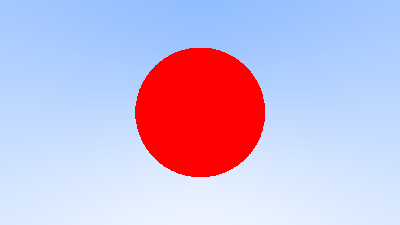# Raytracing in pure shell

## Introduction

This project is still a work in progress and so is this article.

It’s been a long desire of mine to one day write a raytracer. It was even the pet project I was supposed to do in order to learn OCaml in 2020 but I never went as far as to actually install OCaml. Such is life.

But then I read the excellent Ray Tracing in pure CMake and was sufficiently convinced I should not wait any longer and write my own, but in slightly less impractical language: the UNIX shell.

Following the advices on Ray Tracing in One Weekend my goal is to go the furthest without becoming mad.

There are many difficult steps needed in order to be able to achieve this task:

• Calculating with Real numbers ;
• Computing the square root of an arbitrary number ;
• Generating random numbers ;
• Operating on vectors.

I tackled the first two in Fixed point arithmetic in pure shell and Calculating the square root of a number in pure shell so let see what is next.

The code is hosted here if you do not want to read the details: https://git.sr.ht/~tleguern/krt

## Generate PPM images

The second section of Ray Tracing in One Weekend is § Output an Image so I did the same choice: the easy PPM text format. This is a good start and I can always switch to a shell implementation of PNG later.

``````krt() {
cat <<EOF
P3
\$width \$height
255
EOF
j=\$(( height - 1 ))
while [ "\$j" -ge 0 ]; do
for i in \$(\$enum 0 \$(( width - 1 ))); do
r=\$i
g=\$j
b=63
printf "%d %d %d\n" \$r \$g \$b
done
j=\$(( j - 1 ))
done
echo ""
}
``````

A 256x256 sized image takes one minute and forty seconds on my computer, a Thinkpad X1 Carbon 5th. This is going to get worse.

## Vectors

The section three is § The vec3 Class which describes a vector class in C++ and its various functions.

I implemented a very similar looking class in a standalone file to be used as a library by my raytracer. It allows to add, subtracts, multiply and divide between vectors (`vec3_add`, `vec3_sub`, `vec3_mul` and `vec3_div`), multiply and divide a vector with a real number (`vec3_mulf` and `vec3_divf`) as well as various other operations (`vec3_square` or `vec3_sum`).

Lessons learned from Fixed point arithmetic in pure shell multiplications and divisions are implemented using a scaling factor:

``````vec3_mulf() {
local v1x="\$1"; shift
local v1y="\$1"; shift
local v1z="\$1"; shift
local f="\$1"

echo "\$(( v1x * f / 1000 )) \$(( v1y * f / 1000 )) \$(( v1z * f / 1000 ))"
}
``````

While the C++ code is easy to read it makes use of operator overloading: it looks cleaner but the complexity is hidden. In my case I would love to hide a bit of complexity but writing in shell is writing mostly unreadable code.

In order to make my code easier to debug I tend to break long computations in their simpler parts. As a drawback it becomes even harder to grok.

``````local tmp1=\$(vec3_squared \$v1x \$v1y \$v1z)
local tmp2=\$(vec3_sum \$tmp1)
local tmp3=\$(_sqrt \$tmp2)
vec3_divf \$v1x \$v1y \$v1z \$tmp3
``````

In another language such code could be much simply written as `v / sqrt(sum(squared(v)))`.

## Rays

Section 4 § Sending Rays Into the Scene finally adds rays to the raytracer, rejoice.

The whole point is to start using the vector library in order to calculate the color of each pixel on the screen, using rays each time. A ray is actually the combination of two vectors: the point of origin and the direction. In C++ as well as in many languages it is possible to differentiate between these two types of vectors, perhaps by implementing sub-classes or using aliases. There are no practical way of doing that in shell, leading to complicated functions with tons of parameters. In order to reduce future errors I decided to idiot-proof my functions’ parameters handling:

``````ray_idiot_proofed() {
if [ \$# -eq 2 ]; then
local origin="\$1"; shift
local direction="\$1"; shift
else
local origin="\$1 \$2 \$3"; shift 3
local direction="\$1 \$2 \$3"; shift 3
fi
``````

Using this pattern it is not a problem if `ray_idiot_proofed` is called using either `ray_idiot_proofed "\$origin" "\$direction"` or `ray_idiot_proofed \$origin \$direction`. Of course it is still possible to invert the order of the parameters but there is nothing I can do about it.

## Sphere

Nothing much to say here apart of the functions getting uglier and uglier:

``````hit_sphere() {
if [ \$# -eq 3 ]; then
local center="\$1"; shift
local origin="\$(echo "\$1" | cut -d ' ' -f 1-3)"
local direction="\$(echo "\$1" | cut -d ' ' -f 4-6)"
shift
else
local center="\$1 \$2 \$3"; shift 3
local origin="\$1 \$2 \$3"; shift 3
local direction="\$1 \$2 \$3"; shift 3
fi

local oc="\$(vec3_sub \$origin \$center)"
local a="\$(vec3_dot \$direction \$direction)"
local b="\$(( 2000 * \$(vec3_dot \$oc \$direction \$origin) / 1000 ))"
local discriminant="\$(( b * b - 4 * a * c ))"
[ \$discriminant -gt 0 ]
}
``````

## Current resultInterestingly there is a wild difference in performances between the shells for generating this image:

Name Time
dash 24m58s
OpenBSD ksh 47m26s
bash 84m50s
zsh error
yash error

## Changelog

• 2021-01-13: Initial write-up ;
• 2021-01-21: English translation, fleshing up, links to other articles.